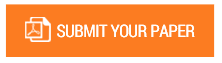On the Classical Derivation of Electrodynamic Equations from the Stationary Action

Authors : Ismail A. Buliyaminu

Volume/Issue : Volume 4 - 2019, Issue 8 - August

Scribd : https://bit.ly/2kGmScq

The Principle of Stationary Action is extremely useful and plays a central role in deriving physics equations. One of the demanding aspects of this topic, difficult to explain, is how it connects with electrodynamic equations. This paper presents a simple derivation of classical electrodynamic equations based on Stationary action principle in which the Lagrangian formalism of a nonrelativistic mechanical system is extended to obtain the relativistic Lagrangian equation of a free particle in an external field. Lorentz invariance and appropriate action integrals for the moving particles in static fields, moving fields, and matter-field interaction are constructed to obtain the equations of motion of charged particles. Inhomogeneous Maxwell’s equations of the electromagnetic field are obtained using electromagnetic field tensor with six independent components of electric field (E) and magnetic field (B) in matrix form via the electromagnetic Lagrangian density. It is also considered that the homogeneous part and the Bianchi identity are derived by introducing a dual field tensor. The continuity equation of motion is presented by introducing electromagnetic 4-divergence.

Keywords : Electrodynamics, Maxwell’s Equations, Bianchi Identity, Lorentz Force And Least Action Principle.

#### CALL FOR PAPERS

Paper Submission Last Date
31 - August - 2022